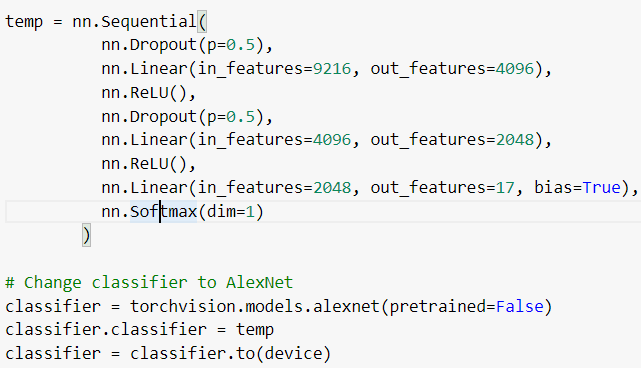# Softmax outputing 0 or 1 instead of probabilities

I am using a pre-train network with nn.BCEWithLogitsLoss() loss for a multilabel problem. I want the output of the network as probabilities, but after using Softmax, I am getting the output of 0 or 1, which seems quite confusing as Softmax should not output perfectly 0 or 1 of any class, it should output the probabilities for various classes instead.

Below is the image of my code:Below is the image of the output:

The softmax operation might output values (close to) this discrete values, if a particular logit in the input activation has a relatively positively large value as seen here:

``````x = torch.randn(1, 10)
out = F.softmax(x, dim=1)
print(out)
> tensor([[0.1612, 0.1486, 0.1232, 0.0626, 0.0162, 0.3084, 0.0166, 0.0811, 0.0098,
0.0723]])

x[0, 1] = 1000.
out = F.softmax(x, dim=1)
print(out)
> tensor([[0., 1., 0., 0., 0., 0., 0., 0., 0., 0.]])
``````

If you are using `nn.BCEWithLogitsLoss`, I assume you are working on a multi-label classification use case. If that’s the case, you should remove the softmax and pass the raw logits to this criterion, as internally `log_sigmoid` will be applied.

Hi @ptrblck,
Yes, it is a multi-label classification problem. Is there a way to convert logits into probabilities, as the softmax is output 0 and 1 for all the observations.
I want to use cutoff point to choose the labels instead of topk classes, what should I do to convert the output into probabilities? As I have tried to take the exp of the logits, but their sum is substantially greater than 1.
Shall I use some different loss to get the probabilities?

For a multi-label classification you would apply `sigmoid` to the outputs to get the probability for each class separately.
Note that `nn.BCEWihtLogitsLoss` still expects raw logits.
You could apply the `sigmoid` and use `nn.BCELoss` instead, but this would reduce the numerical stability.

Using BCELoss throughs an error:

While the same network works with nn.BCEWihtLogitsLoss

One of the tensors (model output, target or weight) is a `DoubleTensor`, while a `FloatTensor` is expected, so you would have to transform it via `tensor = tensor.float()`.